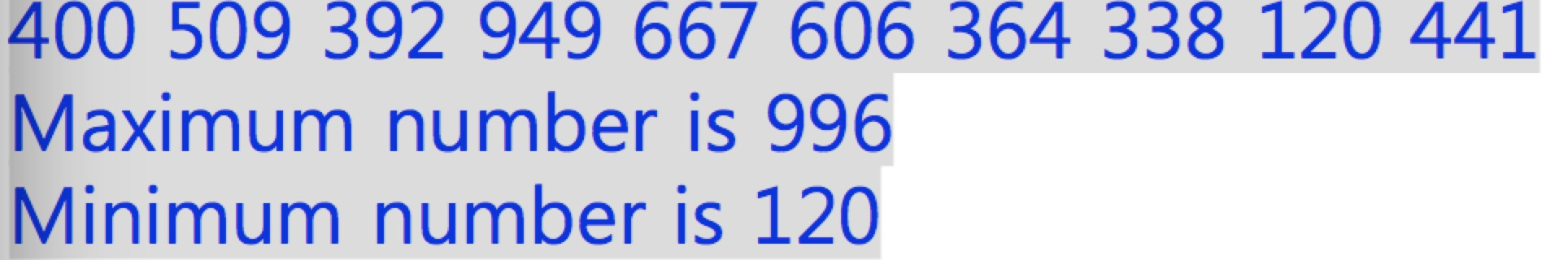2015-10-04 11:27

# c语言编程问题 求大神解答

Maximum number
• Write a program that outputs maximum and minimum number in 10 random numbers
• Range of the random number is 1-1000 • Use the for loop

400 509 392 949 667 606 364 338 120 441 Maximum number is 996
Minimum number is 120• 写回答
• 关注问题
• 收藏
• 邀请回答

#### 4条回答默认 最新

•觅不凡 2015-10-06 03:48
已采纳

本题答案@caozhy 已经实现了，这里贴出一点点优化方案，主要关注以下问题：
1，range范围的变更不需要修改代码其他部分（解耦）
2，for循环内局部变量移出来，因为range范围在整个执行过程中没有变更，没必要每循环一次就创建一次，同样value值也是（性能考虑）

本答案意为讨论所用，有什么问题请多多指正。

``````#include <stdio.h>
#include <stdlib.h>

int main()
{
srand((unsigned)time(NULL));
int range_low = 1, range_high = 1000;  // range范围
int max = range_low, min = range_high;

int value;

int i;
for (i = 0; i < 10; i++)
{
// range [range_low, range_high]
value = (rand() % (range_high - range_low + 1)) + range_low;

if (max < value)
max = value;
if (min > value)
min = value;
printf("%d ", value);
}

printf("\n");
printf("Maximum number is %d\n", max);
printf("Minimum number is %d", min);
return 0;
}
``````
打赏 评论
•tonigthdream 2015-10-04 12:49

class Program
{
static void Main(string[] args)
{
Random objRandom = new Random();
List listNums = new List();
for (int i = 0; i < 10; i++)
{
}
listNums.Sort();
Console.WriteLine(string.Join(" ",listNums.ToArray()));
Console.WriteLine("Maximum number is:{0} Minimum number is {1}",listNums,listNums);
}

打赏 评论
•threenewbee 2015-10-04 17:23
`````` #include <stdio.h>
#include <stdlib.h>

int main()
{
srand((unsigned)time(NULL));
int i;
int ma = 1; int mi = 1000;
for (i = 0; i < 10; i++)
{
int a = 1; int b = 1000;
int r = (rand() % (b - a + 1)) + a; // [a, b]
if (ma < r) ma = r; if (mi > r) mi = r;
printf("%d ", r);
}
printf("Maximum number is %d\n", ma);
printf("Minimum number is %d", mi);
return 0;
}
``````
打赏 评论
•ffiirree 2015-10-06 01:57
`````` #include <stdio.h>
#include <stdlib.h>
#include <time.h>

int main()
{
srand((unsigned)time(NULL));                    /* srand()给rand()提供种子seed，使两次运行产生不同的随机数       */

int max = 1,min = 1000;                         /* 用来保存随机数里的最大最小值，且赋值以便后面的比较存储值     */

for (int i = 0; i < 10; i++)
{
int a = 1,b = 1000;

int r = (rand() % (b - a + 1)) + a;         /* rand()%n会产生0~n-1之间的随机数，这个公式产生a~b之间的随机数 */

if (max < r) max = r;                       /* 得到最大值                                                   */

if (min > r) min = r;                       /* 得打最小值                                                   */

printf("%d ", r);
}

printf("\nMaximum number is %d\n", max);
printf("Minimum number is %d\n", min);

return 0;
}
``````
打赏 评论